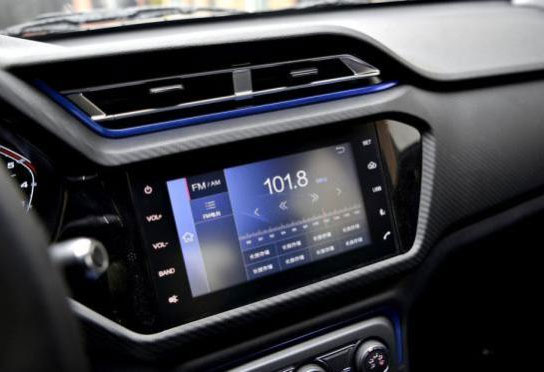## 6万元自动挡SUV 瑞虎3x完胜长安CS15

2017年09月13日 17:33 来源：车行天下 超过：15436次关注

当年轻一代渐渐步入社会，事业通途也开始一路向前。前行路上需要一位亲密小伙伴相助？一款潮流时尚且兼具性价比的车便是理想之选。今天小编就从颇受年轻人喜爱的小型SUV着手，从市场上受欢迎程度最好的车型中，精选了两款6万元出头自动挡的小型SUV，并将两款小车进行全面的对比，为年轻消费者的购车选择提供参考。经过一番筛选，最终锁定在瑞虎3x、长安CS15这两款车型。之所以选择这两款车向年轻朋友推荐，是因为这两款车口碑好，外观时尚、品质过硬，而且都具备6万多元价位的自动挡车型。

首先，瑞虎3x定位跨界运动型SUV，拥有4G-wifi、智能互联，车型也动感时尚， 在小型SUV里已经成为“网红”。长安CS15上市时间比瑞虎3x略早几个月，销量成绩也不错，如何选？我们做了以下对比：

（一）车型对比如上图所示，两款车自动挡起步价格都是6万+，长安CS15的自动挡起步车型价格稍高一些，瑞虎3x有7款自动挡配置车型，所以价格区间稍大。而车身尺寸方面，瑞虎3x以绝对优势领先，对驾驶和乘坐空间都会更宽敞舒适一些。

（二）外观设计对比

外观是进行选择的第一眼要素。瑞虎3x采用了奇瑞“Life in motion”设计语言，强调整体设计的年轻与动感。配备了这个级别的车型并不多见的日间行车灯，而且在前脸格栅、雾灯边框等多处加入了镀铬装饰，看起来很精致；前大灯灯组内部配有透镜，远近光均采用这个级别车型常用的卤素光源。

长安CS15外观也属运动化设计，整体风格比瑞虎3x更为方正。从车身比例、视觉感受都很流畅，唯一的美中不足是线条略显繁复。（三）内饰对比

内饰的设计同样要符合年轻消费者审美。购买这种年轻小车的消费者，绝对不会被前卫大胆的内饰设计所吓倒，两款车均投其所好，做的很出彩，颜色搭配很鲜艳。同样采取三幅式方向盘和两侧采用圆形出风口。整体设计长安CS15比较常规，瑞虎3x则完整地秉承了CHERY DESIGN---H.D.S设计风格，采用不对称设计，突显年轻个性。高配均有中控触摸屏，两款车的中控屏幕分辨率和灵敏度在这个价位都算不错的，但中控屏能给我们带来多少乐趣？作为年轻一代消费群体，大家对智能互联是有较高需求的。长安CS15搭配7英寸触控屏幕，带有导航、蓝牙、音乐等功能，支持內建WiFi以及百度Carlife手机互联，导航采用高德地图。瑞虎3x的8英寸中控大屏配备4G-WiFi，搭载 Cloudrive2.0智云互联行车系统，完全是高级别“城会玩”，娱乐、音乐、导航、生活指南、违章提醒、车辆管理等都可轻松实现，语音识别还可识别多地方言，车载Wi-Fi最多可连接10部移动设备，数据库信息支撑的内容提供商包括大众点评、高德导航、携程旅行网、网易云音乐等；另外多媒体系统还提供手机屏幕映射功能，iPhone或安卓手机都支撑屏幕映射；此外每月可以免费使用500M流量。是不是真的需要这么强大、这么酷？当然。CS15的7英寸中控屏

（四）动力对比

两款车均采用了1.5L自然吸气发动机，长安CS15采用5速湿式双离合变速箱，瑞虎3x搭配的是4AT，二者动力参数并没有太大差距。

油耗方面，瑞虎3x凭借着ECO模式和换挡提醒加上整车低风阻系数，提高经济性，油耗低于长安CS15。而油耗也是年轻消费者考虑的重要因素，一方面的使用成本，另一方面也关乎节能环保。

底盘配置上，两款车都采用前麦弗逊、后扭力梁的悬挂形式，都属于这个级别SUV正常的配置，达到合资小型SUV水平。经过一番比较，不难发现：在潮流时尚和运动感、人性化体验、品质细节方面瑞虎3x做得更好，很符合年轻消费者的style；动力、操控方面两者各有所长；在科技配置方面，瑞虎3x更加走心，智能互联的“黑科技”之强大也更得年轻人喜爱。

最近购买的话，正值奇瑞20周年全系大促，购买瑞虎3x不仅可享受两年24期0利率金融政策，更有超低首付2成起或超低日供22元起的灵活选择，是非常难得的出手机会。

#### 相关文章

0-500 字已有评论 0条 查看评论>>

﻿
• 快速找车
• 选择品牌
• 选择品牌
• A  奥迪
• A  阿斯顿·马丁
• A  阿尔法·罗密欧
• B  宝沃
• B  布加迪
• B  巴博斯
• B  保时捷
• B  宾利
• B  奔驰
• B  宝马
• B  本田
• B  别克
• B  标致
• B  比亚迪
• B  宝骏
• B  北汽制造
• B  北汽新能源
• B  北汽幻速
• B  北汽威旺
• B  北京汽车
• B  奔腾
• B  北汽绅宝
• C  长安
• C  长安商用
• C  长城
• C  昌河
• D  大众
• D  道奇
• D  DS
• D  东南
• D  东风风神
• D  东风风行
• D  东风小康
• D  东风风度
• D  东风
• F  福特
• F  丰田
• F  菲亚特
• F  法拉利
• F  福田
• F  福迪
• F  福汽启腾
• G  观致
• G  广汽传祺
• G  广汽吉奥
• G  GMC
• H  红旗
• H  汉腾汽车
• H  哈弗
• H  哈飞
• H  海格
• H  海马
• H  华颂
• H  黄海
• H  华泰
• H  恒天
• J  吉利汽车
• J  捷豹
• J  Jeep
• J  江淮
• J  江铃
• J  金杯
• J  九龙
• J  金旅
• K  凯翼
• K  凯迪拉克
• K  克莱斯勒
• K  科尼塞克
• K  卡威
• K  开瑞
• L  路虎
• L  林肯
• L  劳斯莱斯
• L  兰博基尼
• L  雷克萨斯
• L  铃木
• L  雷诺
• L  理念
• L  力帆
• L  莲花汽车
• L  猎豹
• L  路特斯
• L  陆风
• M  马自达
• M  MG
• M  MINI
• M  玛莎拉蒂
• M  摩根
• M  迈凯轮
• N  纳智捷
• O  欧宝
• O  讴歌
• O  欧朗
• Q  奇瑞
• Q  起亚
• Q  启辰
• R  日产
• R  荣威
• R  瑞麒
• S  三菱
• S  斯威汽车
• S  萨博
• S  smart
• S  斯柯达
• S  斯巴鲁
• S  思铭
• S  双龙
• S  上汽大通
• S  双环
• T  特斯拉
• T  腾势
• W  沃尔沃
• W  五菱汽车
• W  五十铃
• W  威兹曼
• W  威麟
• X  现代
• X  雪佛兰
• X  雪铁龙
• X  西雅特
• Y  一汽
• Y  英菲尼迪
• Y  英致
• Y  依维柯
• Y  野马汽车
• Y  永源
• Z  众泰
• Z  中华
• Z  中兴
• Z  知豆
• 选择车系
• 选择车系
• 车型对比
• 选择品牌
• 选择品牌
• A  奥迪
• A  阿斯顿·马丁
• A  阿尔法·罗密欧
• B  宝沃
• B  布加迪
• B  巴博斯
• B  保时捷
• B  宾利
• B  奔驰
• B  宝马
• B  本田
• B  别克
• B  标致
• B  比亚迪
• B  宝骏
• B  北汽制造
• B  北汽新能源
• B  北汽幻速
• B  北汽威旺
• B  北京汽车
• B  奔腾
• B  北汽绅宝
• C  长安
• C  长安商用
• C  长城
• C  昌河
• D  大众
• D  道奇
• D  DS
• D  东南
• D  东风风神
• D  东风风行
• D  东风小康
• D  东风风度
• D  东风
• F  福特
• F  丰田
• F  菲亚特
• F  法拉利
• F  福田
• F  福迪
• F  福汽启腾
• G  观致
• G  广汽传祺
• G  广汽吉奥
• G  GMC
• H  红旗
• H  汉腾汽车
• H  哈弗
• H  哈飞
• H  海格
• H  海马
• H  华颂
• H  黄海
• H  华泰
• H  恒天
• J  吉利汽车
• J  捷豹
• J  Jeep
• J  江淮
• J  江铃
• J  金杯
• J  九龙
• J  金旅
• K  凯翼
• K  凯迪拉克
• K  克莱斯勒
• K  科尼塞克
• K  卡威
• K  开瑞
• L  路虎
• L  林肯
• L  劳斯莱斯
• L  兰博基尼
• L  雷克萨斯
• L  铃木
• L  雷诺
• L  理念
• L  力帆
• L  莲花汽车
• L  猎豹
• L  路特斯
• L  陆风
• M  马自达
• M  MG
• M  MINI
• M  玛莎拉蒂
• M  摩根
• M  迈凯轮
• N  纳智捷
• O  欧宝
• O  讴歌
• O  欧朗
• Q  奇瑞
• Q  起亚
• Q  启辰
• R  日产
• R  荣威
• R  瑞麒
• S  三菱
• S  斯威汽车
• S  萨博
• S  smart
• S  斯柯达
• S  斯巴鲁
• S  思铭
• S  双龙
• S  上汽大通
• S  双环
• T  特斯拉
• T  腾势
• W  沃尔沃
• W  五菱汽车
• W  五十铃
• W  威兹曼
• W  威麟
• X  现代
• X  雪佛兰
• X  雪铁龙
• X  西雅特
• Y  一汽
• Y  英菲尼迪
• Y  英致
• Y  依维柯
• Y  野马汽车
• Y  永源
• Z  众泰
• Z  中华
• Z  中兴
• Z  知豆
• 选择车系
• 选择车系
• 选择车型
• 选择车型
• 意见反馈